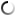# Friday, December 04, 2009

## ColorBarBoing

/**
* by Evan Rakob AKA PixelPusher
* // http://pixelist.info
**/

// push the mouse to see it in action

// this will be the lag variable
float drag = 0.0;

int maxRows = 2;
int maxCols = 40;

void setup()
{
size(480, 270);
colorMode(HSB);
drag = 0.0;

maxRows = 2;
maxCols = 40;
}

// When the mouse is pressed, jump our lag variable to its high value
void mouseReleased()
{
drag = 6.0;
}

void draw()
{
background(80);  // clear screen to gray

noStroke();

// every time we draw, decrease our lag variable by some percent (the higher the value,
// the slower the decrease
drag *= 0.92;

// the easy way:
//int mx = (int) (drag * map(mouseX, 0, width, 1, 30));

// a bit more straightforward:
int mx = 4 + (int) (maxCols * drag * (float)mouseX/(float)width + 1);
float interval = (float)width/mx;

for (int b=0; b < maxRows; b++)
{

// draw some alternating lines on the screen
for (int c=0; c < mx; c++)
{
if (b % 2 == 0)
fill(190.0 * c/(mx-1), 220, 200);
else
fill(190.0 - 190.0 * c/(mx-1), 220, 200);

rect(c*interval, b*height/maxRows, interval, (b+1)*height/maxRows);
}
}
}

## commentsloading...